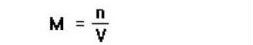## What is Molarity in Chemistry?

Main Learning Goal and Core Concepts: The goal of this lesson is to learn about the factors that influence molarity and how to calculate it.

## Vocabulary

#### Molarity

Molarity is a measure of concentration of a solution. It is defined as the number of moles of solute divided by the volume of the solvent in liters. Molarity varies with temperature and pressure.

#### Solution

A solution is a type of homogeneous mixture made up of two or more substances. At minimum, it is composed of a substance, the solute, dissolved in another substance, the solvent.

#### Solute

solute is the material that is dissolved in a solution. The solute can be a solid, liquid, or gas depending on the solution. It is typically the smaller amount in a solution.

#### Solvent

A solvent is the material that dissolves the solute in a solution. It is usually a liquid, but can also be a solid or a gas. The quantity of solute that can be dissolved varies with temperature.

## Lesson Brief

Before molarity, there are solutions:

A solution is composed of a solvent and one or more solutes mixed together.

• The solvent is the dissolving agent
• The solute is the substance(s) dissolved

Now, what is molarity?

Molarity is a measure of concentration of a solution (not the only measure). It is defined as the number of moles of solute divide by the volume of solution in liters.What are factors that influence molarity?

There are gas laws developed towards the end of the 18th century named by several scientists that predict changes in gases based on changing volume, pressure, and absolute temperature.

• Boyle’s Law which connects the pressure and volume of a gas
• Charle’s Law which connects the volume of a gas and absolute temperature in Kelvin
• Gay-Lussac’s Law which provides a relationship between the pressure of a gas exerted on its container and the absolute temperature

When combined together, the Combined Gas Law shown below reveals that volume is inversely proportional to pressure and directly proportional to absolute temperature.Looking at the molarity equation shown below, molarity is inversely proportional to volume.• When pressure goes up, volume goes down and molarity goes up. Thus, we can conclude molarity is directly proportional to pressure.
• When absolute temperature in Kelvin goes up, volume goes up and molarity goes down. Thus, we can conclude molarity is inversely proportional to volume.Notes: